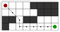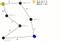+1

# A* Search Algorithm

## What is A* Search Algorithm?

A* (pronounced as "A star") is a computer algorithm that is widely used in pathfinding and graph traversal. The algorithm efficiently plots a walkable path between multiple nodes, or points, on the graph.

## How it works?

Imagine a square grid which possesses many obstacles, scattered randomly. The initial and the final cell is provided. The aim is to reach the final cell in the shortest amount of time.## Explanation

A* works by making a lowest-cost path tree from the start node to the target node. What makes A* different and better for many searches is that for each node, A* uses a function f(n)f(n) that gives an estimate of the total cost of a path using that node. Therefore, A* is a heuristic function, which differs from an algorithm in that a heuristic is more of an estimate and is not necessarily provably correct.

A* expands paths that are already less expensive by using this function:

`f(n)=g(n)+h(n)`

• f(n) = total estimated cost of path through node nn
• g(n) = cost so far to reach node nn
• h(n) = estimated cost from nn to goal. This is the heuristic part of the cost function, so it is like a guess.

## Pseudocode

``````function reconstruct_path(cameFrom, current)
total_path := {current}
while current in cameFrom.Keys:
current := cameFrom[current]
total_path.prepend(current)

// A* finds a path from start to goal.
// h is the heuristic function. h(n) estimates the cost to reach goal from node n.
function A_Star(start, goal, h)
// The set of discovered nodes that may need to be (re-)expanded.
// Initially, only the start node is known.
// This is usually implemented as a min-heap or priority queue rather than a hash-set.
openSet := {start}

// For node n, cameFrom[n] is the node immediately preceding it on the cheapest path from start
// to n currently known.
cameFrom := an empty map

// For node n, gScore[n] is the cost of the cheapest path from start to n currently known.
gScore := map with default value of Infinity
gScore[start] := 0

// For node n, fScore[n] := gScore[n] + h(n). fScore[n] represents our current best guess as to
// how short a path from start to finish can be if it goes through n.
fScore := map with default value of Infinity
fScore[start] := h(start)

while openSet is not empty
// This operation can occur in O(1) time if openSet is a min-heap or a priority queue
current := the node in openSet having the lowest fScore[] value
if current = goal
return reconstruct_path(cameFrom, current)

openSet.Remove(current)
for each neighbor of current
// d(current,neighbor) is the weight of the edge from current to neighbor
// tentative_gScore is the distance from start to the neighbor through current
tentative_gScore := gScore[current] + d(current, neighbor)
if tentative_gScore < gScore[neighbor]
// This path to neighbor is better than any previous one. Record it!
cameFrom[neighbor] := current
gScore[neighbor] := tentative_gScore
fScore[neighbor] := gScore[neighbor] + h(neighbor)
if neighbor not in openSet

// Open set is empty but goal was never reached
return failure
``````

## Example

An example of an A* algorithm in action where nodes are cities connected with roads and h(x) is the straight-line distance to target point:Key: green: start; blue: goal; orange: visited